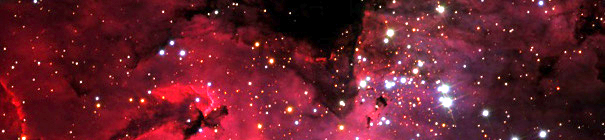Gregory J. Parkerhome simulations scripts et cetera eye contact

Introduction

All of the programs on this page are written in FORTRAN 77/95 and have successfully run on Hewlett-Packard (HP-UX) workstations, IBM (AIX), Linux (Red Hat, Ubuntu, Mandriva), Windows (NT and above) and Mac OS X (including pre-10.0). Typically Intel fortran or gfortran work fine. Historically, Absoft, IBM and HP compilers also present no problems.

While I wish I could upload the source files directly to this server, my ISP would then insist on an additional monthly payment. Since I'm offering these programs free, one can easily see this would be a negative net gain- something I wish not to embark on.

To get around this, you can simply contact me and we'll work out some arrangement.

Program Descriptions

Convected Scheme

This program, unlike the others described here, is actually a set of programs. These programs come in two flavors: self-consistent and field specified. In the self-consistent versions, the CS calculates the electron and ion energy distributions by solving the Boltzmann equation and Poisson's equation simultaneously. Field specified versions solve for only the electron energy distribution in user specified fields in such a way that Vaslov's equation is exactly satisfied. Both versions come in 1d2v (infinite parallel plate) or 1d3v (cylindrical). Electron-electron Coulomb collisions are approximated. Arbitrary electron-neutral collisions with arbitrary differential cross sections are also supported. The numerical algorithm is based on Green's functions. References can be found in the following papers: 1, 2, 3, 4, 7, 8, 13, 16 and 18

This program calculates the temporal and spatial excited resonant state atom density and spectral shape and intensity as a function of an arbitrary (both temporal and spatial) production of excited state atom density and geometry. Current geometries supported are infinite parallel plate, infinitly long cylindrical and spherical. Both complete (CFR) and partial (PFR) frequency redistribution in each geometry is available. For PFR, either Jefferies-White approximation or the angle averaged exact redistribution can be used. Currently, the simulation assumes one symmetric lineshape (Doppler, Voigt or Lorentz) and uniform density of ground state atoms. Transient and steady-state solutions of resonance atom densities are easily computed. The method of calculation is based on Green's functions and is 50 to 100 times faster than equivalent Monte Carlo algorithms. References can be found in the following papers: 5, 6 and 12.

Download tar and gzip'ed source and manuals for ver. 1.2 (73,336 bytes).

Electron Kinetics via Monte Carlo

This program calculates the electron energy distribution function (EEDF) as a function of user specified geometry, fields and collision processes using a Monte Carlo algorithm. Geometries currently supported are 0d3v, 1d3v (either infinite parallel plate or cylindrical geometry) and 2d3v (either Cartesian or cylindrical geometry). Time varying electric and magnetic fields are allowed. Arbitrary electron-neutral collisions are user specified and arbitrary differential cross sections are supported. Output from the code includes the EEDF as a function of kinetic energy and spatial position or the full EEDF as a function of velocity.

Download tar and gzip'ed source and manuals for ver. 2.5 (1,355,478 bytes).

Long Mean Free Path Transport

This program computes neutral transport at low pressure where the mean free path is comparable to the system size. Input is the geometry of the system (either 2d Cartesian or cylindrical) and the source profile of neutrals. Output consists of neutral density as a function of position and the angular distribution of neutrals into surfaces. References can be found in the following papers: 9, 10 and 11.

Feature Profiler

This program evolves a 2d surface according to user specified fluxes emitted 'far away' from the surface. Both etching and deposition is allowed. As the surface evolves, shadowing is automatically included. User can specify the initial surface and an arbitrary incoming flux and output is the temporal evolution of the surface.

Ion Implantation Model

This program calculates the dose profile, in 3d, of an energetic (~keV) ion beam into a amorphous or crystalline solid. The user can specify the solid, channeling planes, and ion beam composition and angle to the surface. Output consists of the 3d density profile of ions and energy deposition into the solid. The algorithm is based on Green's functions and is typically 50-100 times faster than the equivalent Monte Carlo code. References can be found in the following paper: 15.